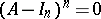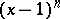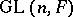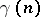# Unipotent matrix

A square matrixover a ring for which the matrix, whereis the order of, is nilpotent, i.e.. A matrix over a field is unipotent if and only if its characteristic polynomial is.
A matrix group is called unipotent if every matrix in it is unipotent. Any unipotent subgroup of, whereis a field, is conjugate into some subgroup of a special triangular group (Kolchin's theorem). This assertion is also true for unipotent groups over a skew-field, if the characteristic of the latter is either 0 or greater than some.